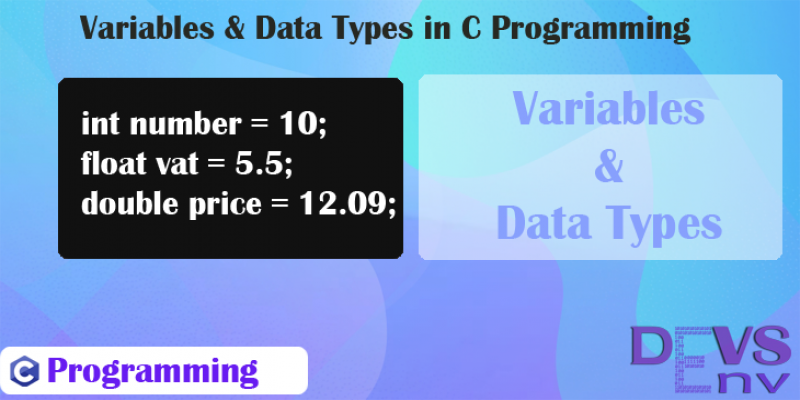Categories - C Programming Tags - C   Maniruzzaman Akash   3 years ago   3263   3 minutes   0

# #2 Variable and Data Types in C Programming

Variable in C is a named location in a memory where a program can manipulate the data. This location is used to hold the value of the variable.

The value of the C variable may get change in the program. C variable might be belonging to any of the data type like int, float, char etc.

We'll walk through this lesson with C programming variables and data types.

What is Variable?

In our mathematical term, variable is something that can be changed.

In Computer Science, variable is a named location in memory where program will handle it's data. We give computer memory location a name, which is used to store data.

What Variable do and Why Variable in Programming?

1. Variables are mainly responsible to store values.
2. It is not possible to remember a memory address and store a value there, but it is easy to make a variable and store data to that memory location.
3. Get value from memory by that variable.

How to Create a variable in C Programming?

Let's see a basic example of variables in C programming.

``````int number1 = 100;
int number2 = 200;
float division = number2 / number1;``````

in this above example of C programming, We've created three variables. Variables are -

1. number1
2. number2
3. division

We can assign value in a variable and we've seen that

1. We've stored 100 as a value in number1 variable. Which data type is integer type (We'll learn that in below section)
2. We've stored 200 as a value in number2 variable.
3. We've stored the division of number2 and number1 in division variable.

We can now access the variables anywhere in our program.

Another Example of Store values in Variable:

``````#include <stdio.h>

int main() {
int age;
float bankAccountBalance;

age = 20;
bankAccountBalance = 200000.50;
}``````

Here are the two variables,

1. age is an integer type variable which can store number type value in memory.
2. bankAccountBalance is a float type variable, which can store decimal values in memory.

Example of Get values from Variable:

Check this example of getting the values from a variable. See the above program fully.

``````#include <stdio.h>

int main() {

// Declare Variables
int age;
float bankAccountBalance;

// Assign / Store values to variable
age = 20;
bankAccountBalance = 200000.50;

// Get values from variable
printf("Your account balance: %.2f BDT\n", bankAccountBalance );
}``````

In the below two lines, We have get the two variables and add a text before printing it.

To access a variable in c, just write this line -

`` printf("%d", age);``

Here, `%d` is for decimal value will be printed, the value would be the `age` value.

We can add extra string before that print, like the above, we've added - `Your age` - extra string. like this -

``printf("Your age: %d\n", age);``

In the second print, look at this code, we've print only the first two after the decimal point.

``printf("Your account balance: %.2f BDT\n", bankAccountBalance );``

That's why the output of this variable would be - 200000.50

Run this simple variable storing and receiving program in C programming language -

Data Types in C Program

Data types in C Programming or in any programming languages is used to define a variables how much data store in memory. It's actually the type of variable. Whenever, we store something in memory, it takes some space from the memory. So, we need to define any variables that how much time it should take from a memory. That's why the data types arrived in C Programming.

C Programming has variaous data types.

Major Data types in C -

1. int             Store integer value
2. float           Store Decimal Numbers, floating point numbers with single precission
3. double        Store Decimal Numbers, floating point numbers with double precission
4. char           Store Single character

All Data types and memory space and other criterias of C Programming -

Data Type Name Memory Storage Range Access Format
int 4 -2,147,483,648 to 2,147,483,647 %d
float  4  -3.4E+38 to +3.4E+38  %f
double  8  -1.7E+308 to +1.7E+308  %lf
char  1  -128 to 127  %c
long int  8  -2,147,483,648 to 2,147,483,647  %ld
long long int  8  -(2^63) to (2^63)-1  %lld
long double  16  -  %lf